# SSAT Middle Level Math : How to find a parallelogram on a coordinate plane

## Example Questions

### Example Question #1 : How To Find A Parallelogram On A Coordinate Plane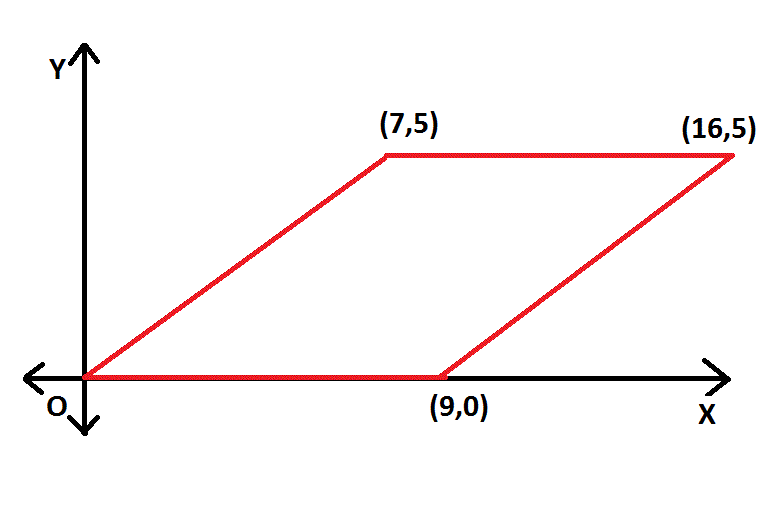NOTE: Figure NOT drawn to scale.

What is the area of the parallelogram represented by the above figure?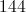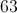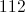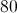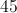Explanation:

The area of a parallelogram is its base multiplied by its height. The diagram below shows both in green: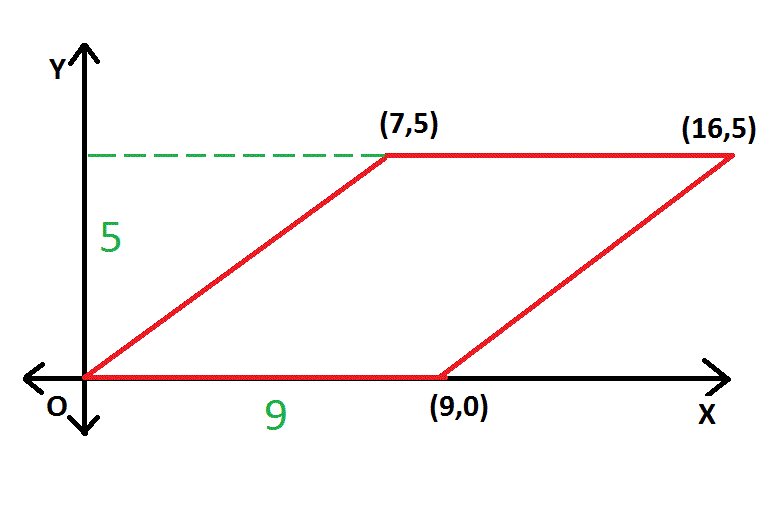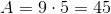### Example Question #32 : Coordinate Geometry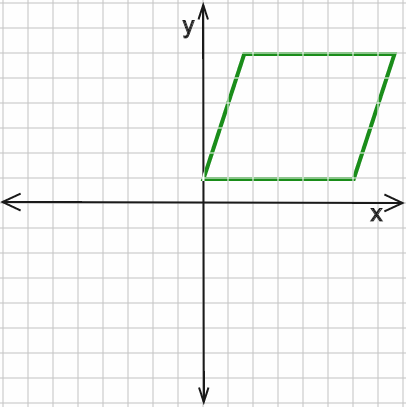Find the area of the above parallelogram given a height of 5 units, as shown to scale.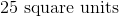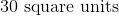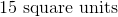Explanation:

Since the diagram is drawn to scale, the base of the parallelogram must be 6 units in length. Using the area formula for a parallelogram: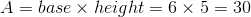### Example Question #1 : How To Find A Parallelogram On A Coordinate PlaneGiven a base of 12 units and an area of 120 square units, find the height of the paralellogram shown above.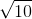Explanation:

The area formula for a parallelogram is: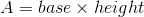Thus, to find the height, rearrange: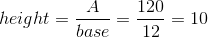### Example Question #2 : How To Find A Parallelogram On A Coordinate PlaneThe above parallelogram has an area of 30 square units. Which of the following could be the lengths of the base and height of this figure? (Image not drawn to scale.)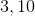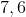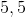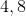Explanation:

The formula for the area of a parallelogram is:Thus, the base and height must equal 30 square units when multiplied together.

3 and 10 are the only combination of base and height which, when multiplied, equal the area of 30 square units.